This function assigns linestring features, many of which in an `sf` object can form route networks, into groups. By default, the function `igraph::clusters()` is used to determine group membership, but any `igraph::cluster*()` function can be used. See examples and the web page igraph.org/r/doc/communities.html for more information. From that web page, the following clustering functions are available:

## Usage

``````rnet_group(rnet, ...)

# S3 method for default
rnet_group(rnet, ...)

# S3 method for sfc
rnet_group(
rnet,
cluster_fun = igraph::clusters,
d = NULL,
as.undirected = TRUE,
...
)

# S3 method for sf
rnet_group(
rnet,
cluster_fun = igraph::clusters,
d = NULL,
as.undirected = TRUE,
...
)

# S3 method for sfNetwork
rnet_group(rnet, cluster_fun = igraph::clusters, ...)``````

## Arguments

rnet

An sf, sfc, or sfNetwork object representing a route network.

...

Arguments passed to other methods.

cluster_fun

The clustering function to use. Various clustering functions are available in the `igraph` package. Default: `igraph::clusters()`.

d

Optional distance variable used to classify segments that are close (within a certain distance specified by `d`) to each other but not necessarily touching

as.undirected

Coerce the graph created internally into an undirected graph with `igraph::as.undirected()`? TRUE by default, which enables use of a wider range of clutering functions.

## Value

If the input rnet is an sf/sfc object, it returns an integer vector reporting the groups of each network element. If the input is an sfNetwork object, it returns an sfNetwork object with an extra column called rnet_group representing the groups of each network element. In the latter case, the connectivity of the spatial object is derived from the sfNetwork object.

## Details

`cluster_edge_betweenness, cluster_fast_greedy, cluster_label_prop,` `cluster_leading_eigen, cluster_louvain, cluster_optimal, cluster_spinglass, cluster_walktrap`

Other rnet: `SpatialLinesNetwork`, `calc_catchment_sum()`, `calc_catchment()`, `calc_moving_catchment()`, `calc_network_catchment()`, `find_network_nodes()`, `gsection()`, `islines()`, `lineLabels()`, `overline_spatial()`, `overline()`, `plot,SpatialLinesNetwork,ANY-method`, `plot,sfNetwork,ANY-method`, `rnet_breakup_vertices()`, `sln2points()`, `sum_network_links()`, `sum_network_routes()`

## Examples

``````rnet <- rnet_breakup_vertices(stplanr::osm_net_example)
rnet\$group <- rnet_group(rnet)
plot(rnet["group"])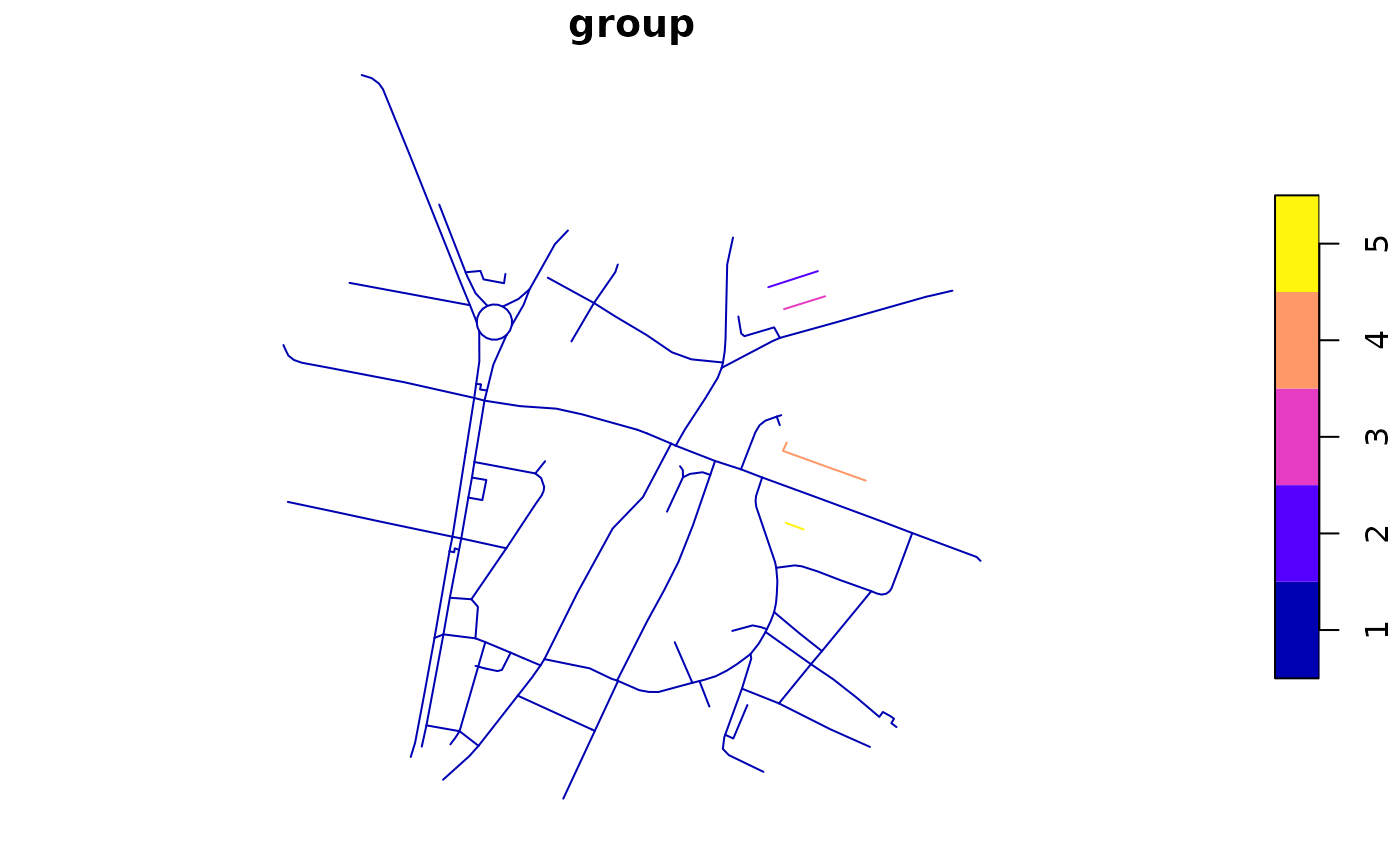# mapview::mapview(rnet["group"])
rnet\$group_25m <- rnet_group(rnet, d = 25)
plot(rnet["group_25m"])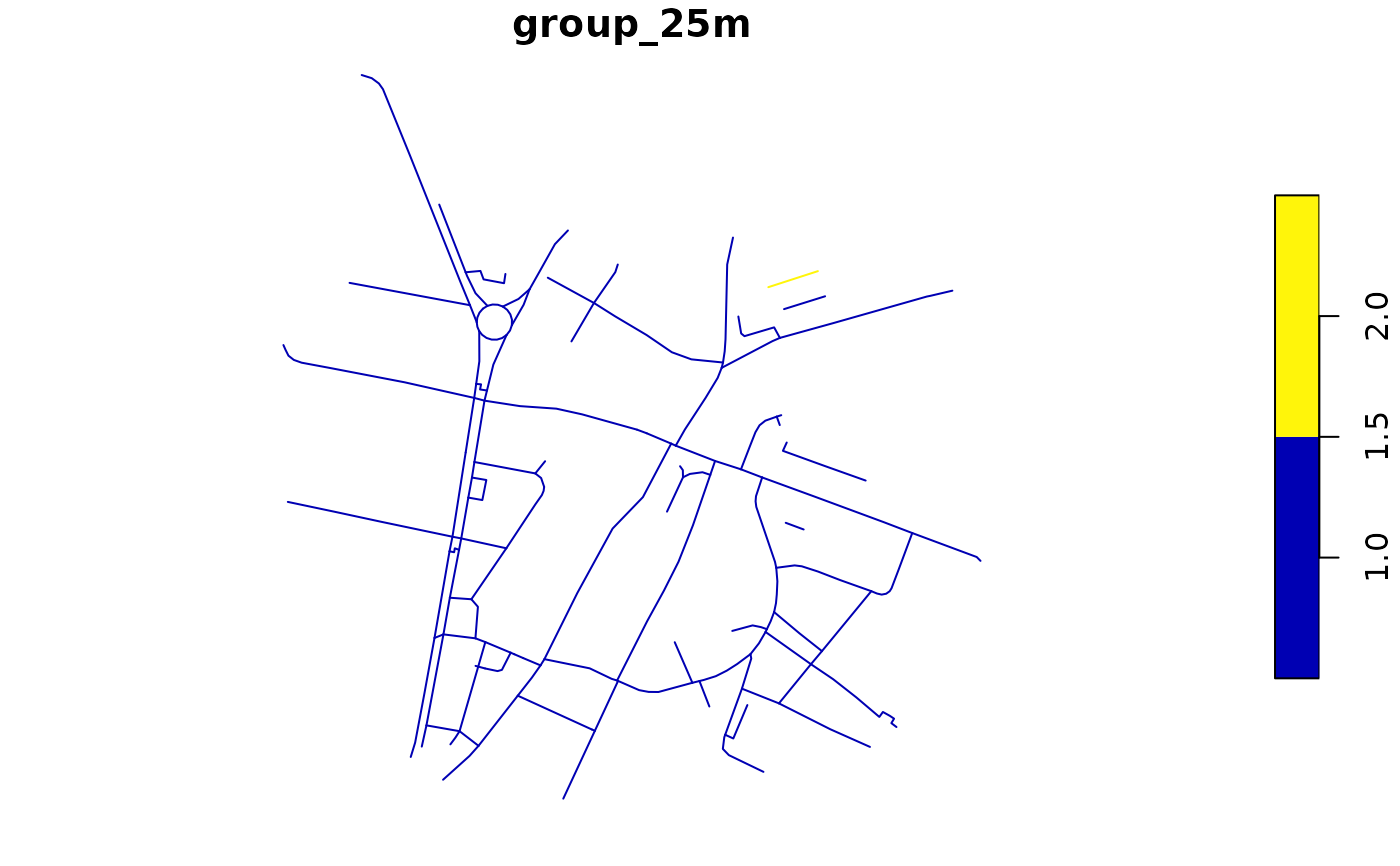rnet\$group_walktrap <- rnet_group(rnet, igraph::cluster_walktrap)
plot(rnet["group_walktrap"])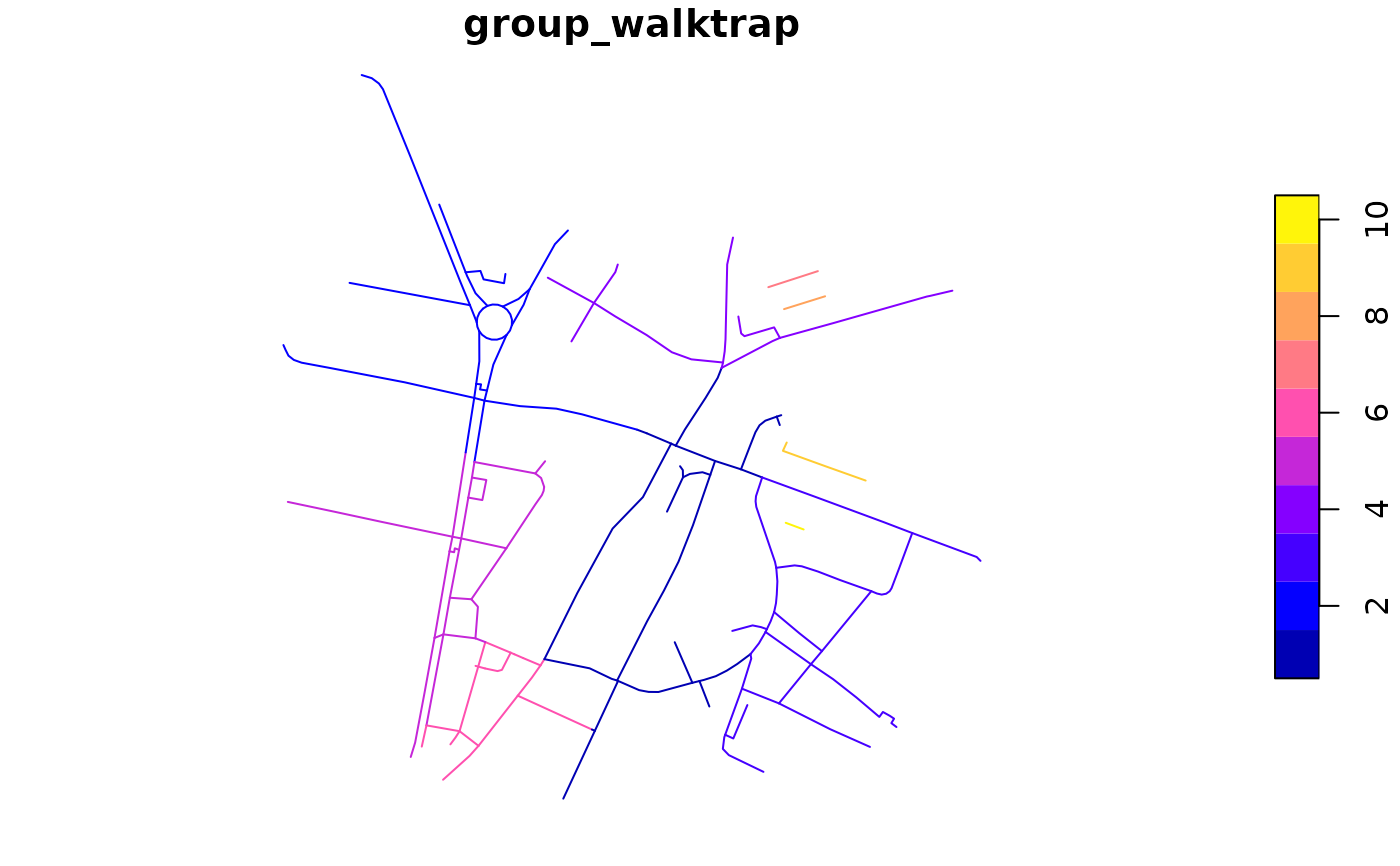rnet\$group_louvain <- rnet_group(rnet, igraph::cluster_louvain)
plot(rnet["group_louvain"])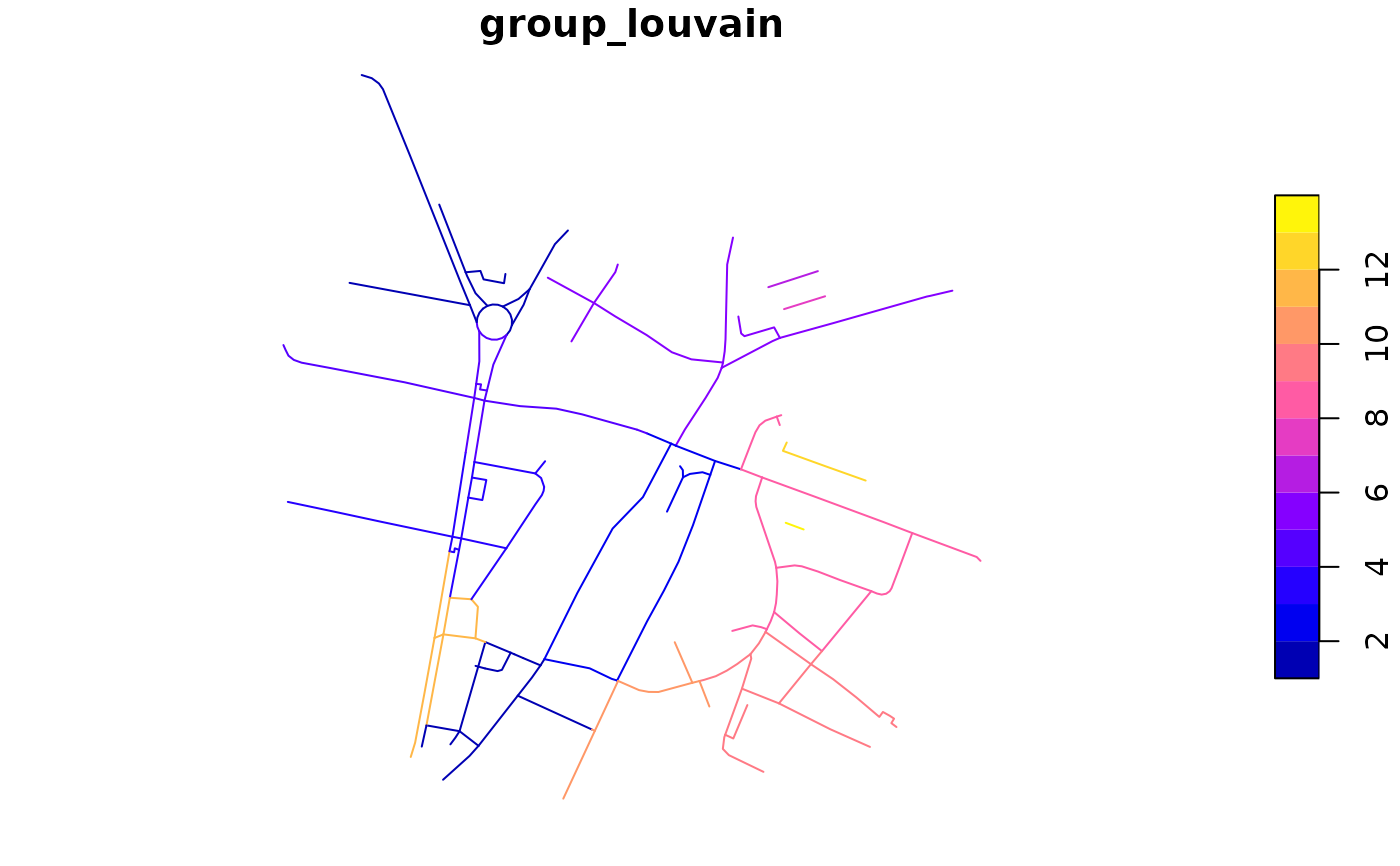rnet\$group_fast_greedy <- rnet_group(rnet, igraph::cluster_fast_greedy)
plot(rnet["group_fast_greedy"])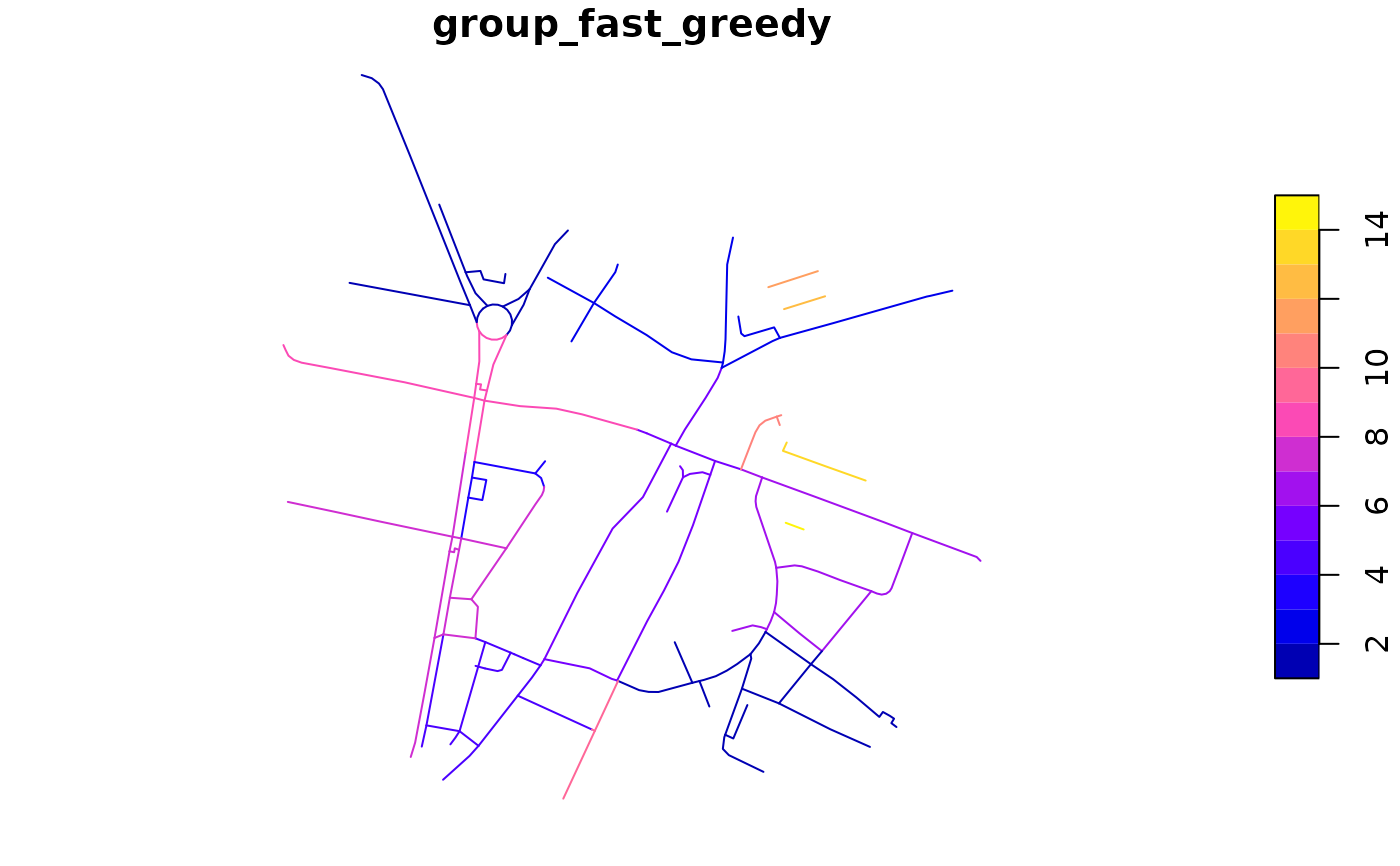# show sfNetwork implementation
sfn <- SpatialLinesNetwork(rnet)
#> Warning: Graph composed of multiple subgraphs, consider cleaning it with sln_clean_graph().
sfn <- rnet_group(sfn)
plot(sfn@sl["rnet_group"])``````